### Nooks and Crannys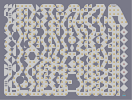Hover over the thumbnail for a full-size version.

Author LHTho0016 author:lhtho0016 autogenerated playable puzzle unrated 2006-11-07 5 more votes required for a rating. \$Nooks and Crannys#LHTho0016#none#99O:=IF25IF25I28@75000695000;AFJMIF:=IF:=0;96IFGH;<5IF2=IF:87P702MI6A6A6AF0KL00;<0>5I6LHGK@7@7@740JM03879669709F25IF:=IF:=I>A?@78690704HG35IF2P78KA?@G34H0666HFHI96LH0GK@?78JIFM6087I=IF:=IF:A>MI>A>9I67015IG;E0>A69F:=0619GH7IF4HG3@0C@?879>78709E0CD0B5IF27969696A>A07E0C87D0G34H0?@?@?@?@?960C=IF:1796198669767998700G;E0>E06LK187LK4HG3LH0GK@0C@0C09000028L7E8171817B78B70000LH000000000000000007400QJ6969696969696969691LH0025252525252525252M111LH34343434343434343L|5^744,558!12^744,480!12^744,432!12^744,384!12^744,336!12^744,288!12^744,240!12^744,192!8^732,564,2!8^756,564,0!8^744,564,1!9^60,228,0,0,3,1,1,0,0!2^36,492,0,-1!2^36,504,0,-1!2^36,516,0,-1!2^60,396,0,-1!2^60,408,0,-1!2^60,420,0,-1!0^684,180!0^684,204!0^684,228!0^684,252!0^684,276!0^684,300!0^684,324!0^684,348!0^684,372!0^684,396!0^684,420!0^684,444!0^684,468!0^684,492!0^684,516!0^684,540!0^588,36!0^564,60!0^540,84!0^516,108!0^516,132!0^540,156!0^564,180!0^552,204!0^540,228!0^540,252!0^564,276!0^564,300!0^564,324!0^540,324!0^540,348!0^564,348!0^564,372!0^540,372!0^540,396!0^564,396!0^564,420!0^540,420!0^540,444!0^564,444!0^564,468!0^540,468!0^540,492!0^564,492!0^564,516!0^540,516!0^540,540!0^588,300!0^588,324!0^588,348!0^612,348!0^588,372!0^588,396!0^588,420!0^588,444!0^588,468!0^588,492!0^588,516!0^588,252!0^588,228!0^588,204!0^564,132!0^564,108!0^564,540!0^564,564!0^588,564!0^588,540!0^660,180!0^660,228!0^660,276!0^660,324!0^660,372!0^660,420!0^660,468!0^660,492!0^660,540!0^708,564!0^708,540!0^708,516!0^708,492!0^708,468!0^708,444!0^708,420!0^708,396!0^708,372!0^708,348!0^708,324!0^708,300!0^708,276!0^708,252!0^708,228!0^708,204!0^708,180!0^708,156!0^636,132!0^636,108!0^540,36!0^516,36!0^516,60!0^492,60!0^492,84!0^492,108!0^492,132!0^468,132!0^492,156!0^492,180!0^516,180!0^516,204!0^516,228!0^516,252!0^516,276!0^540,300!0^516,300!0^516,324!0^516,396!0^516,420!0^516,444!0^516,468!0^516,492!0^516,516!0^516,540!0^684,564!0^660,564!0^636,564!0^612,564!0^612,540!0^636,516!0^636,492!0^612,492!0^612,468!0^636,444!0^636,420!0^612,420!0^612,396!0^636,372!0^636,348!0^636,324!0^612,300!0^612,276!0^636,252!0^636,228!0^612,204!0^612,180!0^612,156!0^588,132!0^588,108!0^612,84!0^612,60!0^612,36!0^540,564!0^516,564!0^492,564!0^468,564!0^468,540!0^492,516!0^492,492!0^468,468!0^468,444!0^492,444!0^492,420!0^468,420!0^468,396!0^492,396!0^492,372!0^468,372!0^468,348!0^492,324!0^492,300!0^468,300!0^468,276!0^492,252!0^492,228!0^468,204!0^468,180!0^444,156!0^444,132!0^444,108!0^468,84!0^468,60!0^468,36!0^684,156!0^660,132!0^684,132!0^660,108!0^660,84!0^660,60!0^660,36!0^684,36!0^684,60!0^684,84!0^708,60!0^708,84!0^732,108!0^732,84!0^756,132!0^732,132!0^444,36!0^444,60!0^420,36!0^420,60!0^396,84!0^396,108!0^372,132!0^372,156!0^420,108!0^420,132!0^420,156!0^420,180!0^420,204!0^420,228!0^444,228!0^444,252!0^420,252!0^396,180!0^396,204!0^372,228!0^372,252!0^396,276!0^396,300!0^372,300!0^372,324!0^396,324!0^420,324!0^444,324!0^444,372!0^444,396!0^420,420!0^444,420!0^396,348!0^372,372!0^372,396!0^396,396!0^396,420!0^372,420!0^372,444!0^396,444!0^396,468!0^372,468!0^420,492!0^372,492!0^396,492!0^372,516!0^396,516!0^396,540!0^396,564!0^372,564!0^348,564!2^60,84,0,-1!2^60,96,0,-1!2^60,108,0,-1!0^324,564!0^324,540!0^324,516!0^300,492!0^300,468!0^324,444!0^324,420!0^300,420!0^300,396!0^324,396!0^324,372!0^348,372!0^348,348!0^324,348!0^324,324!0^348,300!0^348,276!0^348,252!0^324,228!0^324,204!0^300,204!0^300,180!0^324,180!0^324,156!0^300,156!0^300,132!0^324,132!0^324,108!0^300,84!0^300,60!0^324,36!0^300,36!0^276,36!0^372,540!0^348,540!0^348,468!0^348,492!0^348,420!0^348,396!0^348,180!0^348,204!0^348,156!0^348,132!0^348,108!0^372,84!0^348,84!0^348,60!0^372,60!0^372,36!0^348,36!0^300,228!0^300,252!0^324,276!0^300,276!0^300,300!0^300,324!0^300,348!0^300,372!0^276,396!0^276,348!0^276,372!0^252,372!0^252,396!0^228,396!0^228,420!0^252,348!0^252,36!0^252,60!0^276,84!0^276,108!0^252,132!0^252,156!0^276,180!0^276,204!0^252,228!0^252,252!0^276,276!0^276,300!0^252,324!0^276,420!0^276,444!0^276,468!0^252,420!0^252,444!0^228,444!0^228,468!0^252,468!0^252,492!0^276,492!0^276,516!0^252,516!0^252,540!0^252,564!0^228,564!0^204,564!0^276,564!0^300,564!0^180,564!0^228,540!0^228,516!0^228,492!0^204,444!0^204,420!0^228,276!0^228,252!0^228,228!0^180,540!0^204,516!0^204,492!0^180,492!0^180,468!0^156,444!0^156,420!0^180,396!0^204,372!0^180,372!0^156,324!0^228,324!0^180,300!0^204,300!0^180,276!0^180,252!0^204,252!0^204,228!0^180,228!0^180,204!0^204,204!0^204,180!0^180,180!0^180,156!0^204,156!0^204,132!0^180,132!0^180,108!0^204,84!0^204,60!0^180,36!0^156,36!0^228,132!0^228,156!9^228,348,1,0,9,14,1,0,-1!9^156,348,1,0,6,14,1,0,-1!0^156,348!0^228,348!0^156,564!0^132,564!0^156,492!0^156,516!0^132,516!0^156,60!0^156,84!0^156,108!0^156,132!0^156,156!0^156,180!0^156,204!0^156,228!9^60,228,1,1,1,10,0,0,0!9^60,228,1,1,5,2,0,0,-1!11^684,24,60,228!0^132,36!0^108,36!0^132,60!0^108,60!0^108,84!0^132,84!0^132,108!0^108,108!0^108,132!0^132,132!0^132,156!0^108,156!0^108,180!0^132,204!0^132,228!0^108,252!0^108,276!0^132,300!0^132,324!0^108,348!0^108,372!0^132,396!0^132,420!0^108,444!0^84,468!0^84,492!0^108,516!0^108,540!0^108,564!0^84,564!0^60,564!0^36,564!0^36,540!0^60,444!0^36,348!0^60,324!0^60,300!0^36,276!0^36,252!0^60,204!0^36,180!0^36,156!0^60,132# Tunnel of scattered pieces. (FIND THE SIDE ON HEAD, WITH HAT)

## Other maps by this author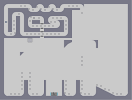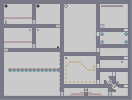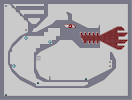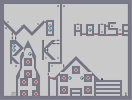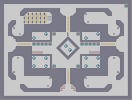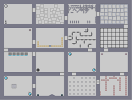Door DDA Rooms N vs. Dragon House and Work Arms and Legs Keep Moving!